## 5.8.1 Window functions

A range of commonly-used window functions may automatically be applied to data as it is read into the fft and ifft commands; these are listed together with their algebraic forms in Table 5.1 and shown in Figure 5.1. In each case, the window functions are given for sample number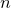, which ranges between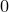and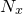. The window functions may be invoked using the following syntax:

fft [...] <out>() of <in>() window <window_name>


Where multi-dimensional FFTs are performed, window functions are applied to each dimension in turn. Other arbitrary window functions may be implemented by pre-multiplying data before entry to the fft and ifft commands.

 Window Name Algebraic Definition BartlettBartlettHann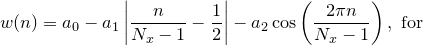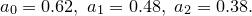Cosine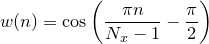GaussHamming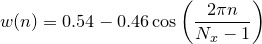Hann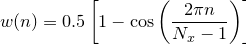LanczosRectangularTriangular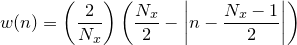Table 5.1: Window functions available in the fft and ifft commands.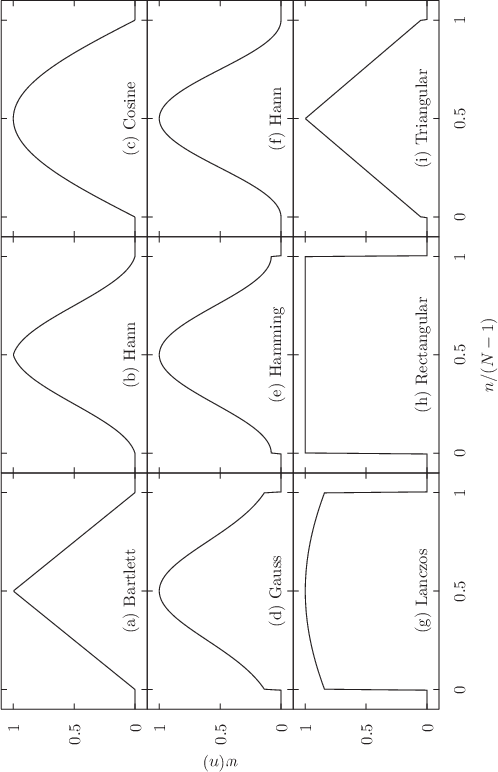Figure 5.1: Window functions available in the fft and ifft commands.